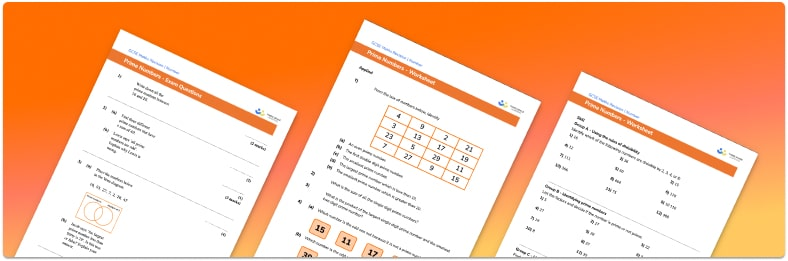# Prime Numbers Worksheet• Section 1 of the prime numbers worksheet contains 20+ skills-based prime numbers questions, in 3 groups to support differentiation
• Section 2 contains 3 applied prime numbers questions with a mix of word problems and deeper problem solving questions
• Section 3 contains 3 foundation and higher level GCSE exam style prime numbers questions
• Answers and a mark scheme for all prime numbers questions are provided
• Questions follow variation theory with plenty of opportunities for students to work independently at their own level
• All questions and maths resources created by fully qualified expert secondary maths teachers
• Suitable for GCSE maths revision for AQA, OCR and Edexcel exam boards

• This field is for validation purposes and should be left unchanged.

You can unsubscribe at any time (each email we send will contain an easy way to unsubscribe). To find out more about how we use your data, see our privacy policy.

### Prime numbers at a glance

Prime numbers are numbers whose only factors are themselves and 1. The prime numbers up to 20 are 2, 3, 5, 7, 11, 13, 17 and 19. Notice that 2 is the only even number which is prime, all other primes are odd numbers. Numbers with more than two factors are called composite numbers. All prime numbers are positive integers.

Every composite number can be written as a product of prime factors. We can use the prime factors of two numbers to find the HCF and LCM of those numbers.

We can use the divisibility rules to determine whether a number is a factor of a given number. If a number has no factors, other than itself and 1, it is prime. The sieve of eratosthenes is another method used for finding prime numbers. It involves using a grid and eliminating all except the first multiple of each whole number in turn.

Looking forward, students can then progress to additional number worksheets, for example a multiplying and dividing decimals worksheet or an order of operations worksheet.For more teaching and learning support on Number our GCSE maths lessons provide step by step support for all GCSE maths concepts.

## Do you have KS4 students who need more focused attention to succeed at GCSE?There will be students in your class who require individual attention to help them succeed in their maths GCSEs. In a class of 30, it’s not always easy to provide.

Help your students feel confident with exam-style questions and the strategies they’ll need to answer them correctly with our dedicated GCSE maths revision programme.

Lessons are selected to provide support where each student needs it most, and specially-trained GCSE maths tutors adapt the pitch and pace of each lesson. This ensures a personalised revision programme that raises grades and boosts confidence.

Find out more